# 4.6 Coercivity of Fe particles with magnetoelastic anisotropyDue to the extreme large plastic deformations originated during the samples cold work, residual stresses can be present in the matrix containing the nanoribbons. These stresses originate lattice distortions that generate an additional term in the energy of the nanoribbons. Clearly, this effect can modify the final coercivity of the nanoparticles. The expression for the magnetoelastic energy is: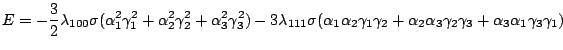(4.3)

whereis the stress magnitude,and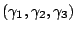are respectively the magnetization unit vector and the cosine director ofin the system of reference given by the lattice axes, andandare the magnetoelastic constant. In the case of the nanoribbons the lattice is rotated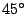with respect to the long axis and supposing that the stress is applied along the long axis of the particles we have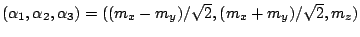. For Fe the magnetoelastic constants areand[Bai 04]. The magnetoelastic energy is then equivalent to an additional uniaxial anisotropy (magnetoelastic) with easy axis in the Y direction and the value:(4.4)

The minimization of magnetocrystalline and magnetoelastic anisotropy in the SW particle gives us the preferred direction of the magnetization for the resulting mixed anisotropy. Fig 4.14 shows the magnetization angle with the long axis of the particle as a function of the stress. The original position of the minimum (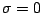) is at. For traction (positive values of) the preferred direction tends to(Y axis) and for compression (negative values of) the preferred direction moves toward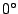(X axis).

In Fig. 4.15 we present our simulational data for a ribbon, including both surface anisotropy (having an effective constant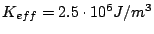) and a magnetoelastic anisotropy arising from uniaxial compression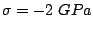. The magnetoelastic anisotropy in this case was directed parallel to the ribbon axis. From the Figure it is clear that this two-parameter (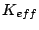and) fitting renders the set of data providing a better description of the experimental ones. Nevertheless, without independent evaluation of at least one of the considered parameter our simulation can not be used to evaluate the second one.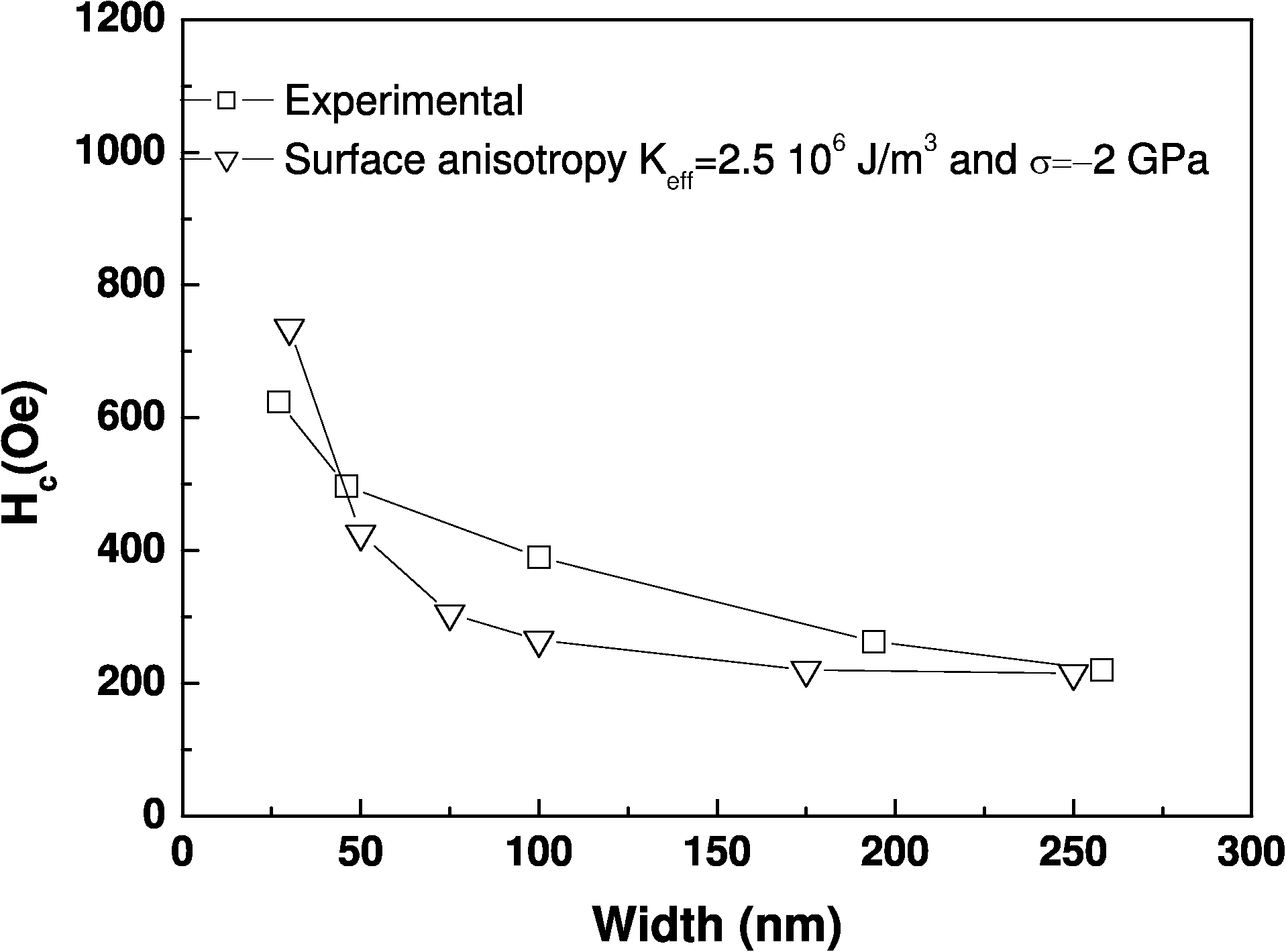2008-04-04